Mathematical Theory and Physical Mechanics for Planetary Ionospheric Physics | OMICS International
Journal of Remote Sensing & GIS

# Mathematical Theory and Physical Mechanics for Planetary Ionospheric Physics

 Jonah Lissner* Independent Researcher, USA Corresponding Author : Jonah Lissner, Ph.D Independent Researcher, USA Tel: +058-142-758-828 E-mail: [email protected] Received January 18, 2015; Accepted June 17, 2015; Published June 24, 2015 Citation: Lissner J (2015) Mathematical Theory and Physical Mechanics for Planetary Ionospheric Physics. J Remote Sensing & GIS 4:148. doi:10.4172/2469-4134.1000148 Copyright: © 2015 Lissner J. This is an open-access article distributed under the terms of the Creative Commons Attribution License, which permits unrestricted use, distribution, and reproduction in any medium, provided the original author and source are credited. Related article at Pubmed, Scholar Google

Visit for more related articles at Journal of Remote Sensing & GIS

#### Abstract

In a dynamic system, e.g., Geometrodynamics geophysical isomorphisms from plasmasphere^i to ionosphere^ii, e.g., Upper-atmospheric lightning (UAL, sferics), Middle-atmospheric lightning and Lower-atmospheric lightning (MAL, LAL, sferics) and Terrestrial and Subterranean Perturbation Regimes (TSTPR, terics) real Physical space is represented as (M,g) R^ 5→(M,g) R^4 brane. F-theory propagates QED continuous polyphasic flux to (Mg) R^4 brane is postulated utilizing Universal constants (K), c.f. Newton's Laws of Motion; c; Phi; Boltzmann's Constant loge S = k W ; Gaussian distributions; Maxwell's Equations; Planck time and Planck Space constants; a; Psi. Constants are propagated from hypothesized compaction and perturbation of topological gauged-energy string landscape (Mg) R^4 d-brane applied to electromagnetic and gravitational Geophysical sweep-out phenomena, e.g. Birkeland currents, ring currents, sferics, terics and given tensorized fields of ionized plasma events^iii and energy phenomena of the near Astrophysical medium. These can be computed from Calabi-Yau manifolds as CP^4 in density matrices of Hilbert space, Hyper-Kahler or 4-Kahler manifolds across weighted projective space. e.g., in Gaussian Unitary Ensembles (GUE) where as a joint probability for eigenvalues and-vectors 3 2 4 1 1 k i j j i k e Z η η λ β βη λ λ − < = Π Π − (1) from dispersion k^2=w^2 p_0 from Boltzmann's constant H  and Trubnikov's 0, 1, 2, 3 tensors [2,3].

 Keywords Newton's laws; Terlezky's quadrigues; Ionospheric physics; Geophysical actions Introduction Constants of dimensional energetics and thermodynamics can be demonstrated as Quantized CEMGD^iv topology of the hypothesized (M,g) R^4 brane. This is proposed to exist at planckscale energetic relationship per qubit formation and QVS/VEV of electronic valence of local stress conditions of (M,g) R^4 brane. Their subsequent discrete Universal for S (Boltzmann, Shannon thermo-entropy) integer value sequences is computed as hyperslices,-surfaces, strings and bundled submanifolds of given phase space timelines. In Geometrodynamic theory^1, Laws of Chaotic Attraction and Nonlinear dynamics, c.f. the theorems of Einstein^2; Wheeler^3; Isham, Butterfield^4; et.al. ^5 are proposed to utilize (M,g) R^4 brane Planck scale. These demonstrate order as formations of entropic phase space events in topological moduli space of instantons and causal structures in conventional (M,g) R^4 brane physics. Aristov has advanced TQFT where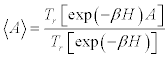, for geometrical orders of Time^5a where R {r ,....,r },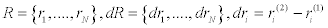i=1,…,N and therefore as Timeentropy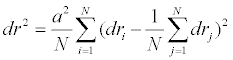Similarly, Universe Mapping has been utilized for WMAP^6, as a standard list of subatomic particles have been discovered at CERN which indicate accurate local predictions of Higgs field and MSSM for Universal redshift and inflationary characteristics ^7. The conventional Ionospheric Electro gravi dynamic (EGD) Energetics Cycle^8 of Dmitriev and Dyatlov's research, is generally inclusive to the fundamental logic of surface-Space calculus, e.g. for a Magnetosonic wave where in general formula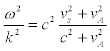, and axioms of formalized, classical Laws of Thermodynamics^9 from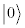as a heterogeneous expression of Thermodynamics and Planck constant and to UCQAIEMGDS^10. The general inequality formula for CEMGD thermodynamics for a single or many-body system can be demonstrated in the universal formula for Bekenstein's Bound^11, where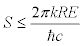. EGD Energetics Cycle is therefore proposed to be in concordance with the Zeroth Law of Thermodynamics^12 as an equivalence relationship where phase space transformation of Energy. This relationship is formulated by the Author (Jonah Lissner) where total energy=fluctuation of temperature and entropy of "closed system" where in general form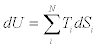or schematically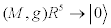where Expectation values of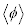for any virtual particles can be measured ΔEΔt≥h. Terlezky's Quadrigues in EGD Physics^8 as developments of Maxwell's extensive Quadratic Matrices can consist of mass, spin or torsion, charge and magnetic moment in a model of interactions between Physical Vacuum, Baryonic Density and Anti baryonic density Universes utilizing Manifold topological paradigm and Manifold Timescales as a capacitor-transmitter. Dyatlov's polarization model of the inhomogeneous vacuum regarding magnetic moments and angular momentum^13 or color charge can be referred in Einstein-de Haas effect^14 where r << M and in Barnett's Effect^15 where r→M and where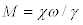. Plasma phenomena such as antimatter have been studied in thunderstorm activity by Briggs, et al. ^16. In actions of purely magnetic or theoretical monopolar measures as B in the EGD schema in R^4 being scalable are approached in formula notation EM and GS inclusive of EGD systemic of energy transformation of gravispin^8: "the electro-magnetic energy converts into heat (EM¤H); the electro-magnetic energy-in the mechanical energy (EM¤M); the electro-magnetic energy in the gravispin energy (EM¤GS); heat - in the gravispin energy (H¤GS); the gravispin energy-in the mechanical energy (GS¤M); the mechanical energy - in heat (M¤H)". Dmitriev and Dyatlov value mass, electrical charge, spin and magnetic moment in chiral, positive and anti-material wavefunction values^8. Regarding energetic measurements of LSS and Astrophysical anomalies in EGD QVS/VEV-Dirac Sea-Brane Boundary-Dark Energy flow confluence of the VD-PV-QVS/VEV-ZPF is generally measured by PE or density of electrons; PG or density of magnetic moments; PM or density of gravitons; and PS or density of Spinors, as polarizations of Vacuum Domains^17. Kerr solitons have been studied in quantum Maxwell effects^18. Rapoport, et al. have proposed phase analysis parameters for ground-to-ionosphere infrasonic waves for earthquake and other terrestrial anomaly prediction engineering^18a. Tomography of radio transmission and IR emission from geological strata (quartz) are proposed to indicate general signals for subterranean perturbation precursors and regimes from ELF>SLF>ULF>VLF or ~3 Hz to 30 kHz or cycles per second. ^18b^18c VLF signals have been indicated as precursors for earthquakes and Vp/Vs for timeline sunset emission measurement^18d^18e. Praxis It is therefore introduced by the Jonah Lissner in an advancement of Planck constant theory regarding integral tensor calculations of PT or density of chrononics as Time-entropy of given discrete causal structure (Hilbert space), as wave functions fromare longitudinal and Spinors. In BDMST Beta decay cycles of plasmatic shell baryonic density and EM dynamism in Hadronic mechanics^19 are generally transverse in orientation. It follows such that solitonic fluctuations of Manifold state energy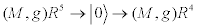brane require by topological origin point an anisotropic, torsional and 4+1 helical relationship. The causality boundary of scalar, longitudinal origination or central Time orientation for T emanation can be established in given isomorphic (M,g) R^4 brane Space-Time fiber-bundles. These bounded complex domains can be defined as the dimensional phase-state and the basis for the integrity of the given dimensional state phase space, e.g. Time dilation in GTR/STR and Field effects for massive bodies as noted in gravitational time dilation mechanics^20 where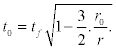(M,g) R^4 brane fiber-bundle activator and Hyperforce node reflection or a dynamic helix in QVS/VEV-Dirac Sea-Brane Boundary- Dark Energy flow confluence of 6 cyclical series (abioplasmatic) energy relationships in BDMST-UCQAIEMGDS are represented in conventional EGD Physics^8 from dipolar PV||APV >> VD >> PVM||PVA. Therefore in sestuplar bracket series as spinor relations and wave propagation EGD: (EM→H EM→M EM→GS H→GS GS→M M→H). ^8 Smirnov has proposed mathematical modeling of ball lightning anomaly as knot fractal strings^20a. In general form in relativistic space-time in (M,g) R^4 brane, the identity E=ML2/T2 holds per Einstein's Strong Equivalence Principle and Energy-momentum relation E2=m2c4+p2c2 thus in Einstein's field effect, the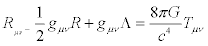transformation can be conjectured where 0 → (M,g) R^4 brane T ≡ E2: EGD. Furthermore for all (M,g) R^4 brane dimension space UCQAIEMGDS Hilbert space topologies N given as subset projection of (M,g) R^4 brane at given QVS/VEV confluence space-time-coordinate per Hasse-Minkowski^21, where furthermore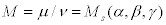and subsequent axes. Bloch-^22 and Neel Walls^23 have been detected using Magnetic anistropy^24; Longo and Iye's research on Universal angular momentum and axial tilt torsion has been demonstrated^25. Generally inertial frequency of all transverse fermionic and bosonic wave functions including longitudinal Lorentz-scalar time wave function chrononic withorigin via supers election from moduli space of instantons and solitonic fluctuation in retro causation. Therefore it is proposed to study these effects per Maxwell's General Equations of Vacuum, also Maxwell's original equations^26 and those equations abridged in vector form^27 computable in surface integrals^28. Photons or pure photonic wave function for mass equivalence of two inertial and gravitational masses^29 where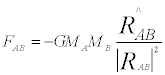, e.g. Sun, Earth and Moon, in general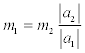. Conditional entropy for two objects can be formulated where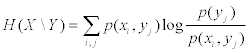. Electronic units in the following table of Maxwell’s Equations in integral or scalar form, are set theoretically for CEMGD to travel as wave functions in longitudinal, transverse and Rayleigh vectors in state of lowest energy approximating zero where given E→(x→,t) and B→ (x→,t):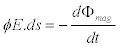Faraday’s Law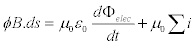Ampere-Maxwell Law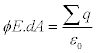Gauss’ Law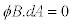Gauss’ Law for magnetism (Maxwell's Equations of Vacuum, Integral Form table, University New South Wales^30)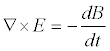(1)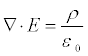(2)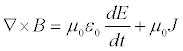(3)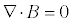(4) (Maxwell's Equations of Vacuum, Differential Form table, University New South Wales^30)(5)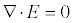(6)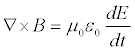(7)(8) (Maxwell's Equations of Vacuum, Velocity of Photon Form table, University New South Wales^30) Therefore postulated to be interacting a revision of the 4 defined Fundamental interactions^31 of the Standard model for the symmetry groups U (1) × SU (2) in Electroweak unification^32 in quaternic interaction of matrices of Special unitary groups^33, in Lie algebra with SU (n) (instants, QCD) for all Physical Constants K in (M,g) R^4 brane in ascending orders of magnitude of solitonic perturbations, as origin points for causation of planetary plasma anomalies. Theory Extrapolations and Predictions for Planetary Ionospheric Physics In BDMST-UCQAIEMGDS topology this is represented as toroidal-spherical or fractal vortex modular decomposition of Scalar Probability-Potential of QVS/VEV, Brane-Bulk Boundary and Knot Compliment with Solitonic Flux Action in Vortical Space, and Gravitational-Pull Of Electronic And Magnetic Fields Of Dense Object in Hausdorff space per BEC^34 actions of gas molecules cooled to 0 K or -273.15 C, where in notation form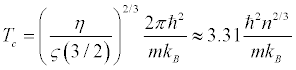. It is therefore proposed radiation of frequency and ionizations of wave function dynamism from moduli space of instantons of proposed QVS/VEV, Brane-Bulk boundary and Knot compliment with solitonic flux action in Vortical spaces across real (M,g) R^4 brane substrate tesselations, e.g., metrics of given system power losses in plasmatic field landscapes such as ionosphere. These occur from Initial state of Cosmic inflation point, hypothetical terms exflation point of (M,g) R^5 hyper point or substrate, in Lorentz Manifold as BDMST postulated reflection space or string vaccua-interchange, as an energy hierarchy described in Physical constants: Strong nuclear force^35 10° Strongest of Standard, (M,g) R^4 brane Forces which does not diminish past 10,000 Newtons or one metric ton, with a distance greater than a Hadron (proton + neutron; boson-baryon_fermion) resulting from gluonic and virtual mesonic attraction at Planckscale. Lapidus and Von Frankenhuysen have advanced arithmetical physics or Quantum geometrics and number theory at this scale for Cantorial fractal strings and spectral Zeta function topology mechanics^36. Weak nuclear force^37 (Leptonic) force 101 Weaker than Strong Nuclear Force, resulting from exchange of virtual particles e.g. Neutrinos, and QVS/VEV fluctuations^38 of W and Z bosons at Plancks scale and has anomalous effects in Quantum tunneling, quantum entanglement and BEC. Electro-magnetic dual or tensegral forces^39 106 Weaker than Weak Nuclear Force, resulting from general Spectrum frequency phase action and interactivity, polyphase dynamism. Anomalies include superconductivity, light-speed fluctuation for engineering. Gravitational force^40 10139 Weaker than Electro-Magnetic Forces, as resulting from electromagnetic wave function dynamics of attraction and dissipation in phase systems of scalar Time-entropy, which vary in coupling force from the minima at CMBR, QVS/VEV, to maxima at Black Holes and Gravastars. Anomalies include superconductivity, periodical element cavitation and micro clustering phenomena; M-theory; Dark energy and gravitational mechanics. In summary, Strong and Weak Nuclear forces are postulated as complimentary and attractive Manifold forces, as are Electronic valence exchange from atomic orbitals and Magnetic attraction fields between said forces in terms of Entropy per accretion velocity and torsion of standing waves. This advances the proposal against the Standard Model^41^42 for Gravitational force as quantumizable in relation to density of estimated nuclear accretions. Rate and entropy of Beta decay as is proposed in MGDS-CEMGD which are demonstrated in dynamic or MHD Plasma domains, e.g., Lightning, sprites, elves, mantles, terrestrial plasma spheres, coronal seismology and superconducting ionospheric phenomena associated with strong high-frequency magnetic reconnection^42a and radiative effects in ionospheric domains^8^17 and terrestrial and subterranean domains^42b. Theory Mathematical Excursion Summary These additional Physical force propositions are introduced by the Author (Jonah Lissner) to present Standard Model, per postulations of BDMST-UCQAIEMGDS theory for Ionospheric physics action: Positive and negative spin-charge Electronic valence-vibratory exchange from atomic nucleus inward-outward wavefunction of torsion of beta decay cycles, et al. as subject to Longitudinal, scalar Magnetic field torsion-resonance of Spinors of atomic matrices in Chrononic wavefunction L-bundle of causation from Scalar Probability- Potential Of QVS/VEV, Brane-Bulk Boundary And Knot Compliment With Solitonic Flux Action In Vortical Space And Gravitational-Pull Of Electronic And Magnetic Fields Of Dense Object of virtual particle and quasi particle perturbation from (M,g) R^5 >> (M,g) R^4. Internal-outward Electronic valence shell exchange from quarks; subatomic nucleus inward-outward wavefunction from nucleus in magnetic matrix at (M,g) R^4. Neutrino exchange from electron positive and negative interchange of valence shell in torsion of beta decay cycles and magnetic matrix of Time and Gravity functions of chrononic and graviton wave functions, at (M,g) R^4. Given Plasmasphere and Magnetosphere perturbations as nonlinear dynamics, and Ionospheric planetary geophysical formations and phenomena are measured by Planck constant polytopic properties and energetics in 4-space, Riemannian constants for spherical celestial bodies, demonstrating hypothesized standing or dynamic, intra orbital Planck constant frequencies in the interstellar medium. These can be demonstrated in Scale-invariance of Titius-Bode laws^43 utilizing major formula variable proofs for scale invariance; Radial velocity to proof of celestial, LSS energetic properties and anomalies; EM frequencies actions and radiation in Plasma dynamics; Rotational velocities of LSS; Orbital velocities of LSS; Angular momentum and QCD color charge of plasma radiation. UCQAIEMGDS morphology and action of orbital correlations in algorithmic function; Gravichronic and interial UCQAIEMGDS morphology and action of orbital correlations in algorithmic function; Gravichronic and interial energy phase space events, e.g. velocity of gravity wave effects and gravitational potentials of Sun >> << Earth^44 where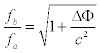. Representation theory for diffeomorphism of groups in Astrophysics^45 where for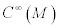and Ix (M) then Diff (M)/Diffx1 (M) can be applied to Geo magnetospheric and Ionospheric metrics^46 and in Gravitational waves from LIGO^47 over Time and Velocity of given phase-space of field. In Quantum mechanics, wave functions can be proposed to regenerate in increasing complexity from standing wave vector scale with origin in Plancks scale. The subatomic and morphology aggregates into molecular polyhedral schema via a^48 or angular measure of Azimuthal Quantum Number^49 where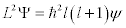. Ratios of Phi operators in electron field gradients and magnetic quadruples, and related domain frequency strength estimations of quantum decoherence of baryonic wave function collapse in aggregations of micro clustering in phase magnitude for given elements. This can be demonstrated in periodic phase of Platinum group inclusive of elemental array series, e.g. Os>Ir>Pt>Au>Hg where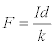per Kirby . Bakhmetyeva, et al. have demonstrated innovative methods for radio-wave resonance scattering to detect chemical anomalies in the Ionosphere^50. It is demonstrated for supposition these phase mechanics pertaining to ion-containment, superconducting, asymmetric gravitation, chrononic-state phase space event qualities and opensystem quantum information^51 hypercube or 3D cell computation engineering, e.g. Electronic Quantum Holography (EQH) ^52 for advances in metrics of Quantum Monte Carlo^53 methods for study of cosmological origin and states of Ionospheric noble gases He, Ne, Ar, et al. in ionic containment and perturbation regimes. Bunzli, et al. has advanced Light-emitting cell (LEC) technology utilizing diffraction techniques of elemental Cl and N gas across Ir lattices . Ionospheric wave function transforms can be demonstrated further schematically as→ (M,g) R^4 brane domain states of electromagneto gravidynamic actions and baryonic density in any given Space-Time node from Zero point state wherein per Planck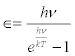from Quantum Harmonic Oscillation (QHO)^54 at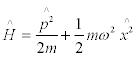. UCQAIEMGDS^10 can be defined as a physical method of domain transformation across scalar, longitudinal Time, torsional, spintronic gravito-electric and topological quantum fields in multiple vectors. Universal physical constants, as well as undiscovered physical laws of Inflationary physics in (M,g) R^4 brane and (M,g) R^5 as physical laws and constants that may exist beyond (M,g) R^5 possible concordance of Dark energy and inflation and Brane theory. The study of the UCQAIEMGDS qualities of quantum thermodynamics is of relevance to prove the existence of generalized laws for engineerable local-scalar time domain states with quantuminformational characteristics: Quantum potential generally has phase space event for solution here in which DBBHSHP quantum decoherence of wave function collapse is postulated as the result of (M,g) R^5→→ (M,g) R^4 brane fiber-bundle scalar Time bipolarity such that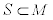via orthagonal, virtual polarization phase space EM moment quantum phase space events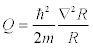. QVS/VEV origin wavefunction or flux behaves as energetic Time timeline tensor set (-CEMGD) of solitonic plasma fluid dynamics in (M,g) R^5→→ (M,g) R^4 brane per Neutronic beta decay model. Cosmological anomalies of this action can be demonstrated in Black holes, sprites,  jets, black or dark aurorae dynamics on or near-Earth [8,9]. Similarly extra planetary plasmatic meteorology, e.g. Great Storm System of Jupiter or spheromak, and jets and shear layers^55 can be formulated where in surface forces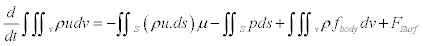and in conservation of energy whereDark baryonic density walls, e.g. Dark energy^56 clusters are proposed as causative, anti-baryonic density refractions fromM-Space coordinates and Killing vectors^57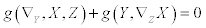or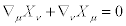and in Bogoliubov transformation^58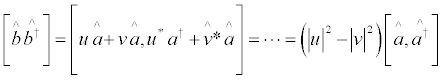. These demonstrate anti-gravitational properties and baryonic density phase space events of positive-exchange electronic wavefunctions having temporal origination in Lobachevskiian QVS/ VEV transformation as (M,g) R^5→→ (M,g) R^4 brane. In the Magnetosphere of the Sun and Earth, Parker has advanced hyperbolic geometry models for the Heliospheric current sheet^58a to advance MHD for plasma electro-magnetics and gravitics^59^59a. It is proposed by the Author (Jonah Lissner) to study the problem of Time-scalar domain recursion in Manifold theory thermodynamically when examining the following dilemma to determine: If (M,g) R^5=anergetic, atemporal vacuum-state domain Space beyond QVS/VEV confluence baryonic density/anti-baryonic density generation, CEMGD and Quantum Probability/Phase Space Events have been diverged from (M,g) R^5 as broken symmetry of particle wavefunctions. These perturbations can point to a Manifold dimension of instanton wavefunction-dynamics in Ionospheric CEMGD. These plasma mechanics computed for algorithms  and a Many-body Schrodinger where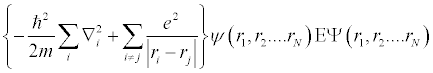These geophysical actions may be realized from active galactic nuclei ^59b or normal galaxy accretion regimes  radiating radio and EM wavelength networks and regions a.cross stellar regions  (IBEX 2009) . Perturbed solar system ionization regimes form solar flares, propagation of UV, radio wave, and lightning-storm dynamics actions through massive geological and atmospheric solid and pervious bodies^60 and can be measured in L-shells^60a where in general formula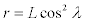Ergodic brane perturbations from (M,g) R^4 universe-phase space event-matrices, or string landscapes of Riemannian curvature at Confluent Hyper force Effect (Jonah Lissner, CHE_1) or Conformal Hyperfield Effect (Jonah Lissner, CHE_2, Z) approximately equivalent to Kretschmann scalar where in general formula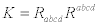. Coleman and de Lucca invoke AdS/CFT to study gravitational effects above Planckscale . Brane-bulk boundary spaces, topological solitons and subsequent modular QVS/VEV confluence quantumtopological probability generation can be demonstrated in correlative effects from stellar and galactic phase currents regimes  on a relativistic scale. (M,g) R^5 →or as in Riemann-Cartan-simple Ricci scalar^61 where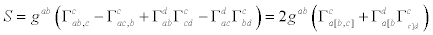. (M,g) R^4 brane curvaturedemonstrated hyperbolically where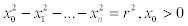, and in the mapping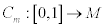. In the geospheric landscape Coleman proposed Q-ball field effects^62 as a result of nontopological bosonic action for QVS/VEV where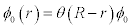and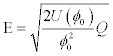Selvam and Fadnavis have proposed prime-adicity in nonlinear and dissipative atmospheric systems where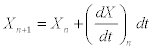, and in general formula for Zeta function analysis, z/lnz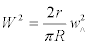and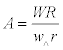[16,17]. The density matrix can be determined therefore where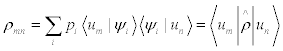. Ionospheric QVS/VEV anomalies and atmospheric plasma densities can be computed as string landscapes  given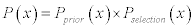in AIT and p-Adic mechanics for UCM where in CPN measure many Quantum finite automata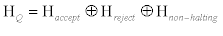for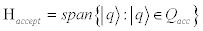and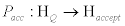to states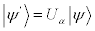where for M p,x set {a, b}* . Therefore a proposed probability model for Kolmogorov structure function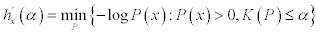where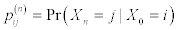. In Ionospheric and Geophysical theory this is proposed for any initial generation of broken symmetry dynamism in Planck constant may have state-vector-virtual or null empirical metrics in (M,g) R^4 brane. This can be composed of present computational models of electronic exchanges with protons or neutron-nucleation, neutrinos>>neutrons of Hydrogen. Therefore are subsequent fermonic, bosonic, baryonic combinations of macro-baryonic density and EM microwave radiation for Hadronic dynamics and solitonic perturbations, e.g., computable heuristics of -CEMGD from planetary Ionospheric physics ( c.f. AMPERE), such as Atmospheric behavior and Earthquake prediction^42b. References Melrose (1997) The response tensor for a highly relativistic magnetized thermal plasma. Journal of Plasma Physics 58: 735-745. Melrose(1997) Covariant form of Trubnikov's response tensor for a relativistic magnetized thermal plasma. Journal of Plasma Physics 57: 479-488. Melrose (1996) Response of relativistic anisotropic thermal plasma. Part 1. Unmagnetized particles. Journal of Plasma Physics 58: 705-719. Watch invisible gravity waves rumble through atmosphere, extracted Kirby BJ (2009) Micro- and Nanoscale Fluid Mechanics: Transport in Microfluidic Devices, Cornell University. Bunzli AM, Constable EC, Housecroft CE, Prescimone A, Zampese JA et al. (2015)Exceptionally long-lived light-emitting electrochemical cells: multiple intra-cation n-stacking interactions in [Ir(C^N)2(N^N)][PF6] emitters. Chemical Science Advance Article 6: 2843-2852. Liu N, Dwyer JR, Stenbaek-Nielsen HC, McHarg MG (2015) Sprite streamer initiation from natural mesospheric structures 6: 7540. Northern lights: How 'black' auroras actually work, extracted. Russell AJB, Karlsson T, Wright AN (2015)Magnetospheric signatures of ionospheric density cavities observed by Cluster, Journal of Geophysical Research, Space Physics. AndersonDV (1989) Parallel algorithms for plasma physics calculations, Computer Physics Reports 11: 117-135. Xiao-xin F, Qiu-sheng G (2010) Measurements of the Stellar Velocity Dispersions of Seyfert Galaxies. Chinese Astronomy and Astrophysics 34: 245-254. Galactic Magnetic Fields May Control Boundaries Of Our Solar System, extracted. Schwadron NA, Allegrini F, Bzowski M, Christian ER, Crew GB et al. (2011) Separation Of The Interstellar Boundary Explorer From Globally Distributed Energetic Neutral Atom Flux. The Astrophysics Journal 731: 1. Coleman S, Luccia FD (1980) Gravitational effects on and of vacuum decay, Physical Review D 21: 12. Alfvén H, Carlqvist P (1978) Interstellar clouds and the formation of stars. Astrophysics and Space Science 55: 487-509. Selvam AM, Fadnavis S (1998) Cantorial Fractal Patterns, Quantum-Like Chaos And Prime Numbers In Atmospheric Flows, IITM. Selvam AM, Fadnavis S (1998)Signatures of a Universal Spectrum for Atmospheric Interannual Variability in Some Disparate Climatic Regimes IITM 66: 87-112. Cvetic M, García-Etxebarria I, Halverson J (2010) On the computation of non-perturbative effective potentials in the string theory landscape -- IIB/F theory perspective. Freivalds(2009)Ultrametric automata and Turing machines 10: 98-112.
Select your language of interest to view the total content in your interested language

### Article Usage

• Total views: 12205
• [From(publication date):
September-2015 - Jan 22, 2020]
• Breakdown by view type
• HTML page views : 8363Can't read the image? click here to refresh